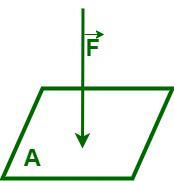GFG App
Open AppBrowser
Continue

# Pressure Conversion Table

Pressure is defined as the force applied perpendicularly to the surface of an object per unit area over which that force is distributed. In simple words, pressure is the ratio of force to area. It is a scalar quantity and is represented as “p or P.” We have various units to express pressure, and some of these are derived from the ratio of the unit of force to the unit of area. The SI unit of pressure is the Pascal (Pa), and apart from it, we also have different units to measure the pressure that are frequently used, such as pounds per square inch (psi), atmospheric pressure (atm), bar, torr, etc. The formula to calculate pressure when a force of “F” Newton is applied perpendicularly to a surface area “A” is equal to the ratio of “F” to “A.”

p = F / A

where,
p” is the pressure exerted on the surface by the force F
F” is the force applied perpendicularly to the surface of an object
A” is the surface area of an objectThe SI unit of pressure is the Pascal, where one Pascal is equal to one newton per square meter.

1 Pascal = 1 N/m2

## How to Use Pressure Conversion table?

A pressure converter table helps in converting one unit of pressure to other units of pressure quickly and easily. The table given is a pressure conversion table that helps convert pressure units.

To use the chart, locate the desired pressure unit and multiply it by the value in the pressure unit column that corresponds to it.

Example: Convert 2 millibars to kilopascal (kpa)

Solution:

To convert millibars to kilopascals we have to multiply 2 by 0.1, which gives the required value in kpa,

i.e.,

2 millibars = 2 × 0.1
= 0.2 kilopascals.

## Solved Examples on Pressure Conversion

Example 1: Convert the 1.5 Kilopascal to Pascal.

Solution:

From the pressure conversion table, we have

1 kilopascal = 1000 pascal

So, to convert 1.5 kilopascals to pascal, multiply 1.5 by 1000, i.e.,

1.5 Kpa = 1.5 × 1000 Pa

1.5 Kpa = 1500 Pa.

Hence, 1.5 Kilopascals is equal to 1500 pascal.

Example 2: Convert the 22 millibars to atm.

Solution:

From the pressure conversion table, we have

1 mbar ≈ 9.869 × 10-4 atm

So, to convert 22 kilopascals to pascal, multiply 22 by 9.869 × 10-4, i.e.,

22 millibar ≈ 22 × 9.869 × 10-4 atm

22 millibar ≈ 0.021712 atm

Hence, 22 millibar is approximately equal to 0.021712 atm.

Example 3: Convert the 0.35 psi to pascal.

Solution:

From the pressure conversion table, we have

1 psi ≈ 6894.757 pascal

So, to convert 0.35 psi to pascal, multiply 0.35 by 6894.757, i.e.,

0.35 psi ≈ 0.35 × 6894.757 Pa

0.35 psi ≈ 2,413.16495 Pa

Hence, 0.35 psi is approximately equal to 2,413.16495 Pa.

Example 4: Convert the 145 kilogram-force/square meters to the bar.

Solution:

From the pressure conversion table, we have

1 kgf/m2 ≈ 0.0000981 bar

So, to convert 145 psi to pascal, multiply 145 by 0.0000981, i.e.,

145 kgf/m2 ≈ 145 × 0.0000981 bar

145 kgf/m2 ≈ 0.0142196425 bar

Hence, 145 kilogram-force/square meters are approximately equal to 0.0142245 bar.

Example 5: Convert the 68 inHg to ft(H2O).

Solution:

From the pressure conversion table, we have

1 inHg ≈ 1.132925 foot of water

So, to convert 68 psi to pascal, multiply 68 by 1.132925, i.e.,

68 inHg ≈ 68 × 1.132925 ft(H2O)

68 inHg ≈ 77.0389 ft(H2O)

Hence, 68 inHg is approximately equal to 77.0389 ft(H2O)

Example 6: What is the value of 5 torrs in pascal?

Solution:

From the pressure conversion table, we have

1 torr ≈ 133.32236 pascal

So, to convert 5 torrs to pascals, multiply 5 by 133.32236, i.e.,

5 torr ≈ 5 × 133.32236 Pa

5 torr ≈ 666.6118 Pa

Hence, 5 torrs are approximately equal to 666.6118 pascals.

## FAQs on Pressure

Question 1: Define pressure.

Pressure is defined as the force applied perpendicularly to the surface of an object per unit area over which that force is distributed. In simple words, pressure is the ratio of force to area.

Question 2: What is the formula to calculate pressure?

The formula to calculate pressure when a force of “F” Newton is applied perpendicularly to a surface area “A” is equal to the ratio of “F” to “A.”

p = F/A

where
p” is the pressure exerted on the surface by the force F,
F” is the force applied perpendicularly to the surface of an object, and
A” is the surface area of an object.

Question 3: What is meant by pascal?

The SI unit of pressure is the Pascal, where one Pascal is equal to one newton per square meter.

1 Pascal = 1 N/m2

Question 4: How does the surface area of an object affect pressure?